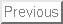Next: Introduction

Sufficient Optimality for Discretized Parabolic and Elliptic Control Problems

Hans D. Mittelmann
Department of Mathematics
Arizona State University
Tempe, AZ 85287-1804, U.S.A.
mittelmann@asu.edu

Abstract:

We study optimal control problems for semilinear parabolic and elliptic equations subject to control and state constraints. We quote known second-order sufficient optimality conditions (SSC) from the literature. Both problem classes are discretized by a finite difference method. The discrete SSC are stated and numerically verified for fine discretizations with the help of sparse linear algebra techniques. This confirms initial results reported earlier for such discretized control problems. In order to relate these results to optimality for the underlying continuous problems corresponding theoretical, especially convergence, results are still unavailable at present.

Hans Mittelmann
2000-08-31